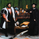# Forecasting - Holt’s Linear Trend Forecastingצפיות ‎2107‎
Holt's Forecasting method

Holt (1957) extended simple exponential smoothing to allow the forecasting of data with a trend. This method involves a forecast equation and two smoothing equations (one for the level and one for the trend):

Forecast equation: ŷ = l + h * b
Level equation: l = alpha * y + (1 - alpha) * (l + b)
Trend equation: b = beta * (l - l) + (1 - beta) * b

where:
l (or l) denotes an estimate of the level of the series at time t,
b (or b) denotes an estimate of the trend (slope) of the series at time t,
alpha is the smoothing parameter for the level, 0 ≤ alpha ≤ 1, and
beta is the smoothing parameter for the trend, 0 ≤ beta ≤ 1.

As with simple exponential smoothing, the level equation here shows that l is a weighted average of observation y and the one-step-ahead training forecast for time t, here given by l+b. The trend equation shows that b is a weighted average of the estimated trend at time t based on l-l and b, the previous estimate of the trend.

The forecast function is not flat but trending. The h-step-ahead forecast is equal to the last estimated level plus h times the last estimated trend value. Hence the forecasts are a linear function of h.
הערות שחרור: Minor fix in documentation.
סקריפט קוד פתוח

ברוח TradingView אמיתית, מחבר סקריפט זה פרסם אותו עם קוד פתוח, כך שסוחרים יוכלו להבין ולאמת זאת. הידד למחבר! אתה יכול להשתמש בו בחינם, אך שימוש חוזר בקוד זה בפרסום הנו בכפוף ל כללי הבית . אתה יכול להגדירו כמועדף ולהשתמש בו בגרף.

רוצה להשתמש בסקריפ זה בגרף?

## תגובותUPD: use f instead of forecast The line forecast = l + b may be removed.
השבRafaelZioni
@RafaelZioni, thx for you suggestion, but I left it as such deliberately as a short-term forecast.
Anyway, you can update in the Inputes section:
ex = input(true, "Extend Right")
And then in the Main section:
fl := line.new(time, close, time + 60 * 60 * 24 * 6, f,
xloc.bar_time, extend=ex ? extend.right : extend.none, style=line.style_solid, color=color.blue, width=2)
השב